View Course Path

# Fault Collapsing methods and Checkpoint Theorem in DFT (VLSI)

Contents

## Fault Reduction

In modern VLSI chips, there are millions of transistors corresponding to millions of interconnecting wires or nets. Each of these nets can potentially exhibit the stuck-at-0 or stuck-at-1 fault. Thus, testing all of these stuck-at faults one-by-one is very inefficient. There can be billions of test vectors!

Luckily, the stuck-at faults in a circuit show a relationship among themselves by virtue of two properties. These relationship parameters help us to reduce our test vectors effectively since instead of targeting individual faults, we can now target groups of similar faults.

There are two essential properties of fault:

• Fault Equivalence
• Fault Dominance

### Fault Equivalence

A single test can detect more than one fault in a circuit, and many tests in a set detect the same faults. In other words, the subsets of faults detected by each test from a test set are not disjoint. A significant objective in test generation is to reduce the total number of faults. This is done by grouping equivalent faults in subsets. It is then sufficient only to test one fault from each equivalent set to cover all the faults. Such subsets are called functionally equivalence classes.

Let’s take an example of a 3-input NAND gate. This gate has 2x(3+1) = 8 stuck-at faults. So, initially, without any optimization, it would require eight different test vectors to detect all the faults. Our task is to minimize the number of test vectors as much as possible.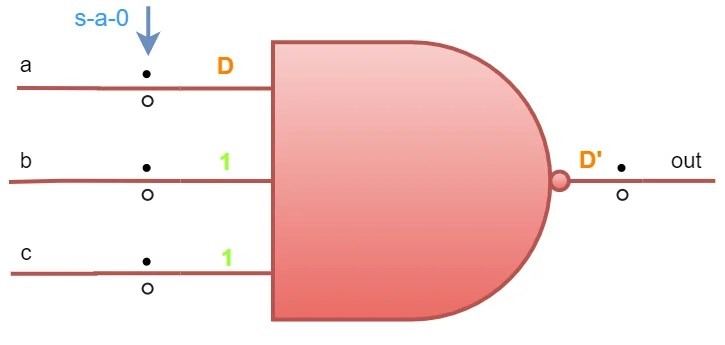The input and output s-a-0 & s-a-1 faults are indicated in the gate schematic. Let’s first generate a test vector for a→s-a-0 at the input. We will use the path sensitization approach. To excite the fault, we need to force logic-1 at the input ‘a’. A discrepancy ‘D’ will be created at ‘a’. Now, to propagate the fault (or ‘D’) to the output (or make it observable), we need all other inputs (‘b’ and ‘c’) as logic-1. So, the test vector for checking a→s-a-0 would be {a, b, c} = {1, 1, 1}. Now, repeat similar steps for ‘b’ and ‘c’ too.

Did you observe? For checking s-a-0 fault at ‘b’ and ‘c’, we again need to apply the same test vector. {1, 1, 1} is a very good test vector as it can check three faults together at once. Hence, we can conclude that s-a-0 faults at the input of any order NAND gate are equivalent.

Can we do it for s-a-1 faults too? Let’s make a table consisting of all input and output stuck-at faults and their test pattern.

 Faults Type Fault Location Test Vector {a, b, c} s-a-0 a {1, 1, 1} b {1, 1, 1} c {1, 1, 1} out {0, x, x} or {x, 0, x} or {0, x, x} s-a-1 a {0, 1, 1} b {1, 0, 1} c {1, 1, 0} out {1, 1, 1}

Unfortunately, we can’t do this for s-a-1 faults because they all need different test vectors.

For out→s-a-0, we just need to excite the fault, no need to propagate, as it’s already at the output and always observable. So, for s-a-0 fault excitation, we need to force out→1. This can be done by applying logic-0 at any of the inputs. Hence, there are multiple test vectors.

For detection of out→s-a-1, it is required to force out→0. This can be only done using the test vector {a, b, c} = {1, 1, 1}. Did you notice something special? Yes, even out→s-a-1 is equivalent to s-a-0 at a, b & c.

 Faults Type Fault Location Test Vector {a, b, c} s-a-0 a, b, c {1, 1, 1} out {0, x, x} or {x, 0, x} or {0, x, x} s-a-1 a {0, 1, 1} b {1, 0, 1} c {1, 1, 0} out {1, 1, 1}

In general, an m-input gate can have a total of (m+1) equivalent sets of faults. We have successfully reduced the test pattern to a great extent. It can be concluded that s-a-0 faults at the input and s-a-1 fault at the output of any order NAND gates are always equivalent.

Two faults f and g are said to be functionally equivalent under a test set T if and only if Z­­­­f(t) = Zg(t) for every test t ∈ T. This technique of reduction of a set of faults to be analyzed based on equivalence relations is called equivalent fault collapsing.

We can collapse the equivalent faults in the gate schematic and keep only one. As they are equivalent, testing only one of them is sufficient. This is a visual representation of fault reduction. By convention, we usually keep the input faults; we will eventually come to know the reason for this later. Below are the reduced table and schematic diagram.

 Faults Type Fault Location Test Vector {a, b, c} s-a-0 out {0, x, x} or {x, 0, x} or {0, x, x} a, b, c {1, 1, 1} s-a-1 out a {0, 1, 1} b {1, 0, 1} c {1, 1, 0}The equivalent collapsed faults are crossed in blue. These equivalent faults can be represented in a special set known as equivalence set.

Equivalence Set = {a-sa0, b-sa0, c-sa0, out-sa1}

This equivalence set consists of four equivalent faults. We can collapse any three of them and retain one. In the above figure, we have retained ‘a‘. We can retain any of the three input equivalents (a-sa0 or b-sa0 or c-sa0}. The best practice is to always collapse the output first.

All the faults in an equivalence set can be tested using only a single test vector.

### Fault Dominance

 Faults Type Fault Location Test Vector {a, b, c} s-a-0 out {0, x, x} or {x, 0, x} or {0, x, x} a, b, c {1, 1, 1} s-a-1 out a {0, 1, 1} b {1, 0, 1} c {1, 1, 0}

Notice that there can be several test patterns for out→s-a-0 in the table. Hence, test constraints on out→s-a-0 are very less. This proves as an advantage, as it can intersect with the test pattern of other faults, further reducing the test vector.From the Venn-diagram, we can notice that out→s-a-0 is dominant to a→s-a-1, b→s-a-1, and c→s-a-1. Note that these aren’t equivalent, we can only choose one test pattern at a time. For the dominant fault (out→ s-a-0), only one test vector is required out of all these possible combinations (blue, red, or green). Hence, even if we are testing any of the blue, red, or green (corresponding to a→s-a-1, b→s-a-1, and c→s-a-1, respectively), the dominant one gets tested automatically.

Therefore, we don’t test dominant faults separately, as they are always tested automatically from test vectors of other faults.

The reduction of the set of faults to be analyzed based on dominance relations is called dominant fault collapsing.

Now, we reduced another test vector. The resulting table and schematic are shown below.

 Faults Test Vector {a, b, c} a→ s-a-0,    b→ s-a-0,    c→ s-a-0,    out→ s-a-1 {1, 1, 1} a→ s-a-1,    out→ s-a-0 {0, 1, 1} b→ s-a-1,    out→ s-a-0 {1, 0, 1} c→ s-a-1,    out→ s-a-0 {1, 1, 0}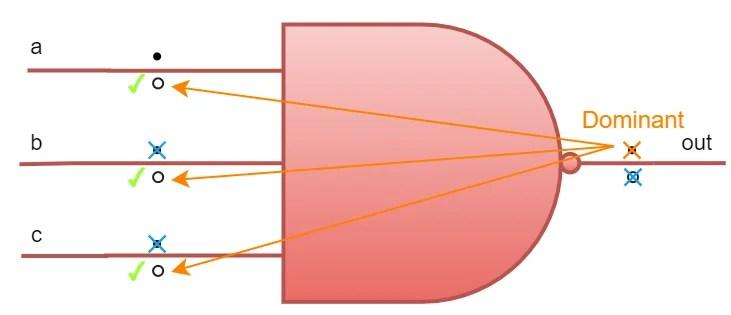The dominant collapsed fault is crossed in orange. The dominance relationship can be represented in another special set known as a dominance set.

Dominance Set = {out-sa0: a-sa1, b-sa1, c-sa1}

The entity before the colon in the set represents the dominant fault, while those after the colon represents its subsets. In dominance relation, we can only collapse the dominant entity (before the colon).

Dominance set with “m” no. of entities after the colon requires “m” no. of test vectors to test the set.

In this case, three test vectors or test patterns {a, b, c} = {(0, 1, 1), (1, 0, 1), (1, 1, 0)} are required to test the above dominance set.

We did a pretty good job in collapsing the faults, straightway reducing eight vectors into four. The test pattern size is now reduced by 50%. The final test pattern required to test all the faults is {a, b, c} = {(1, 1, 1), (0, 1, 1), (1, 0, 1), (1, 1, 0)}

Finally, we conclude that, for any input NAND gate, input s-a-0 faults and output s-a-1 faults are equivalent while output s-a-0 fault is dominant to input s-a-1 faults.

## Fault Collapsing for common gates

In the previous section, we analyzed the equivalence and dominance relations for the 3-input NAND gate. Similarly, analysis can be done for common 2-input logic gates too. I recommend you apply a similar path sensitization method with a tabular approach to figure out the fault relations of these gates and compare your results with the following table.

OR GateNOR GateAND GateNAND GateNOT GateBuffer
 Fault Test Vector {a, b} s-a-0 a {1, 0} b {0, 1} out {x, 1} or {1, x} s-a-1 a {0, 0} b {0, 0} out {0, 0}
Equivalence set: {a-sa1, b-sa1, out-sa1}

Dominance set: {out-sa0: a-sa0, b-sa0}Fault Test Vector {a, b} s-a-0 a {1, 0} b {0, 1} out {0, 0} s-a-1 a {0, 0} b {0, 0} out {x, 1} or {1, x}
Equivalence set: {a-sa1, b-sa1, out-sa0}

Dominance set: {out-sa1: a-sa0, b-sa0}Fault Test Vector {a, b} s-a-0 a {1, 1} b {1, 1} out {1, 1} s-a-1 a {0, 1} b {1, 0} out {0, x} or {x, 0}
Equivalence set: {a-sa0, b-sa0, out-sa0}

Dominance set: {out-sa1: a-sa1, b-sa1}Fault Test Vector {a, b} s-a-0 a {1, 1} b {1, 1} out {0, x} or {x, 0} s-a-1 a {0, 1} b {1, 0} out {1, 1}
Equivalence set: {a-sa0, b-sa0, out-sa1}

Dominance set: {out-sa0: a-sa1, b-sa1}The images in the above tabs are the basis for solving fault collapsing problems on large circuits. Here’s a summary of all the above tabs.

 Gate Equivalence Set(s) Dominant Set(s) OR {a-sa1, b-sa1, out-sa1} {out-sa0: a-sa0, b-sa0} NOR {a-sa1, b-sa1, out-sa0} {out-sa1: a-sa0, b-sa0} AND {a-sa0, b-sa0, out-sa0} {out-sa1: a-sa1, b-sa1} NAND {a-sa0, b-sa0, out-sa1} {out-sa0: a-sa1, b-sa1} Buffer {in-sa0, out-sa0} {in-sa1, out-sa1} null NOT {in-sa1, out-sa0} {in-sa0, out-sa1} null

Don’t worry; you won’t need to memorize the whole table, just memorize the first row (i.e. equivalence and dominant set for OR gate). This table follows the principle of Boolean Duality. Hence, to find the equivalence and dominance sets for AND gate, just replace sa0 with sa1 for both the sets.

For finding for their NOT counterparts, NOR and NAND respectively, just swap the out-sa0 with out-sa1 and vice versa. This is because NOR and NAND gates are just an inverted output of OR and AND gates respectively. And you are smart enough to figure out for NOT gate and Buffer! Note that, for NOT gate and Buffer, there are two equivalence sets, but no dominance relations.

What about XOR and XNOR gates? Let us figure them out too.

XOR Gate
 Fault Test Vector {a, b} s-a-0 a {1, 0} or {1, 1} b {0, 1} or {1, 1} out {0, 1} or {1, 0} s-a-1 a {0, 0} or {0, 1} b {0, 0} or {1, 0} out {0, 0} or {1, 1}

Well, that’s a lot of mess! The reason we are getting two possible test vectors for each fault is that XOR gate never masks inputs. Say for a→s-a-0, we have excited the fault by forcing a→1. Now to propagate fault, we have the freedom to choose ‘b‘ as logic-0 or logic-1.$out\quad =\quad a'b\quad +\quad ab'\quad =\quad D'b\quad +\quad Db'$

D will propagate if b = 0 and D’ will propagate if b = 1. Nonetheless, at every condition, the D will propagate to the output. That’s why we are getting multiple vectors for every possible faults. So how will we decide equivalence and dominance? Let’s count the occurrence of each possible test vectors in the above table.

 Test Vector Fault Coverage {0, 0} 3 {0, 1} 3 {1, 0} 3 {1, 1} 3

In this case, all of the above patterns can detect three faults. Hence every fault is equivalent and dominant to each other. It is difficult to represent any equivalence or dominance relations among themselves. In this case, just choose any three vectors so that fault coverage is 100%.

XNOR Gate

Similarly, the same case can be observed for the XNOR gate too (as we did for XOR gate).

 Fault Test Vector {a, b} s-a-0 a {1, 0} or {1, 1} b {0, 1} or {1, 1} out {0, 0} or {1, 1} s-a-1 a {0, 0} or {0, 1} b {0, 0} or {1, 0} out {0, 1} or {1, 0}

It is noteworthy that,

For any “n” input gate, the number of faults remaining after equivalent and dominant collapsing is “n+1”.

## Fault Collapsing in circuits

Let’s try out a few examples on fault collapsing.

### Fanout-free Circuit

Q. Reduce the stuck-at faults in the following circuit using equivalence and dominance relations.Initially, there are 30 stuck-at faults (2 x No. of wires = 2 x 15) in the gate level schematic. For fault collapsing, we move backwards, parallelly evaluating each gate in each level from the primary output(s) to the primary inputs. Let’s highlight the highest gate-level first.There is only one AND gate. Equivalent faults are: {a-sa0, b-sa0, out-sa0}. We need to test only one fault out of these three. Hence, we collapse two and retain one. Our standard practice is to collapse the output faults first and retain one of the inputs. In this case ‘out‘ is collapsed first. Now, either ‘a‘ or ‘b‘ can be collapsed as per your choice. We collapse ‘b‘ and retain ‘a‘. Equivalent fault collapsing is shown in blue.

Dominance relation for AND gate is: {out-sa1: a-sa1, b-sa1}. Here, out-sa1 is dominant to the other two. Unlike equivalence, in dominant fault collapsing we don’t have any choice, but to collapse the dominant one only. Dominant fault collapsing is shown in orange.

Three faults are collapsed till now, and three remaining. The remaining three faults are considered for collapsing (if possible) in the next lower gate level.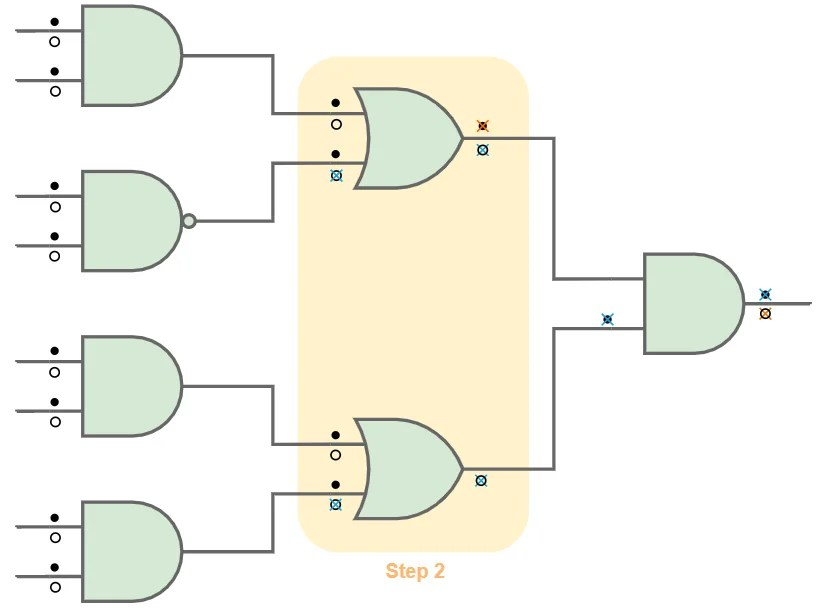The remaining faults are bought closer to the lower level gates (just for simplicity; the stuck-at faults can be represented anywhere in its respective wire). In this level, there are two OR gates. The fault collapsing technique is followed similarly (as in step 1) using equivalence and dominance relations (from the table). For OR gate all s-a-1 faults are equivalent while output s-a-0 is dominant. This way, outputs are collapsed and inputs retained.Same steps are repeated for step 3. Note that there are three AND gates but one NAND gate too. Make sure you apply respective dominance and equivalence relations for respective gates. Note, even if the same faults are collapsed for both the gates, there is a slight difference in which faults are dominant and which are equivalent. This can be clearly seen in the diagram in orange and blue cross notations.

Yay! We have successfully collapsed 18 faults out of total 30 faults. Now, we only need to generate a test pattern for the remaining 12 faults. This is insane!

The collapse ratio is a benchmarking parameter for DFT CAD tools and is defined as no. of remaining faults divided by total no. of faults.

Collapse Ratio = 12/30 = 0.4

The smaller is the collapse ratio, means lesser faults are remaining and hence, better the CAD tool.

Also, notice that the output, as well as all the internal stuck-at faults, are collapsed. We now only need to test the primary inputs. This concludes to the following theorem.

A test set that detects all single stuck-at faults on all primary inputs of a fanout free circuit must detect all single stuck-at faults in that circuit.

### Fanout Circuit

Q2. Reduce the stuck-at faults in the following circuit for minimum collapse ratio, using equivalence and dominance relations.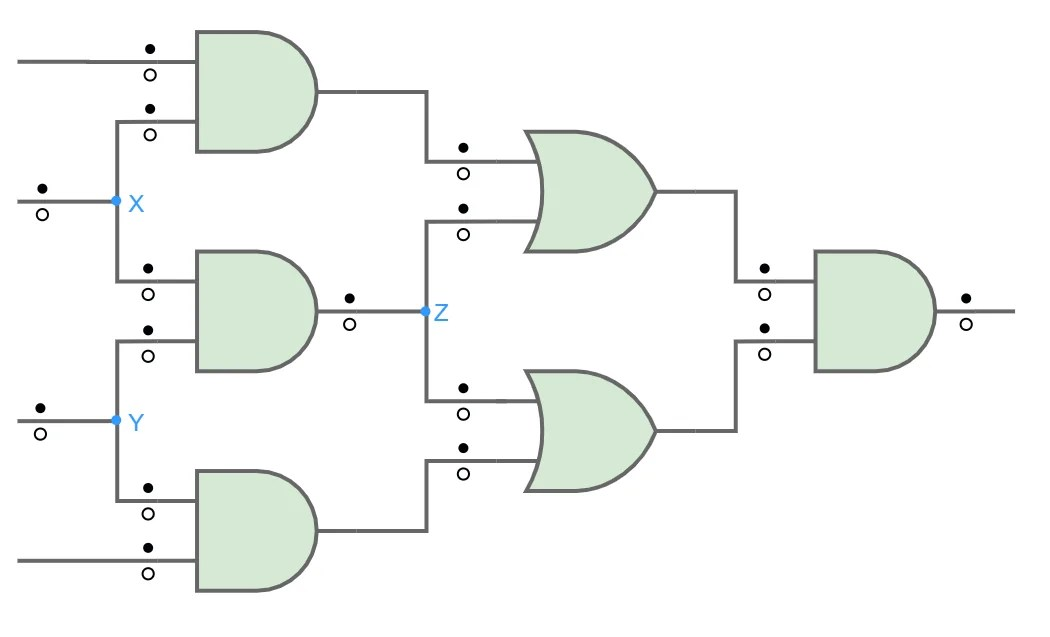The fanout branches (X, Y, Z) are indicated in the circuit.

The stuck-at fault should be considered separately for every fanout branches and even the fanout stem.

As we know that stuck-at faults are just the manifestations of physical defects in transistors, hence each fanout branches and stems represent their respective gate’s transistor defects. For example, the two fanout branches at ‘Z‘ represent faults inside their respective OR gates. Similarly, the fanout stem represents faults in the previous level AND gate. Hence, even being emerging from the same wire, fanout branches and stem must be considered as separate stuck-at faults. Therefore, considering all fanout stems and branches as separate wires, there are 2×16 = 32 total stuck-at faults.

Let’s solve this. But before expanding the solution spoiler, I heavily recommend you to collapse the faults by yourself. This can be done in the same way we did our previous example. There is a very interesting conclusion to draw out based on your solution.

Solution

Come on! Take out your pen and paper. This is going to be an interesting exercise; trust me.

If you are getting, collapse ratio as 15/32 or 16/32 or 17/32, then you are doing all right. Cheers!

But what is happening? Why three different solutions? Why different collapse ratios?

Well, there are numerous ways to collapse a fault; it’s your choice to choose which equivalent fault to retain and which one to collapse. Until now, for fanout free circuit (in the previous example) it was not a problem. But fanout circuits in DFT makes our life difficult.

Fanout branches do not collapse completely. As we can see in all three solutions, fanout branches are not in a mood to collapse. This is a significant problem in DFT. Hence, we need to act smartly.

All three solutions are correct, but the 3rd solution is the best one, as the collapse ratio is least among all three. In the first solution, for OR gate G5 we collapsed b→s-a-1 and retained a→s-a-1. This was the wrong decision. Collapsing b→s-a-1 makes no sense because ‘b‘ is the output of AND gate G3 too. We very well know that for AND gate output s-a-1 fault is dominant. But this fault is collapsed by the limited equivalence relation of OR gate G5. Hence, we didn’t get a chance to utilize dominant fault collapsing. That’s why we don’t have an orange cross across AND gate G3. Notice that fanout branches are the one who is causing the problem. Hence, we should collapse the fanout branches first (i.e. a→s-a-1). We don’t need to bother about other s-a-1 faults, as they will be already collapsed by dominance in the next iteration.

In a fanout circuit, the fanout branches must be collapsed first in equivalent fault collapsing.

The equivalent collapsing direction is shown in blue arrows. For the G5 gate, the arrow is away from the fanout branch of ‘Z‘. This is not as desirable as we discussed above. Notice, for the G4 gate, the collapsing direction is already towards the fanout branch. Hence, we can observe the orange cross, which indicates dominance relations of the G1 gate. This is the desired path.

For the 2nd solution, we did everything wrong, as both the equivalence arrows are pointing away from the fanout branches. This is even worse than the 1st solution, and hence collapse ratio also increases.

3rd solution is the perfect one as both the equivalence arrows are directing towards both the fanout branches. More faults are collapsed, and hence collapse ratio is the least in this case.

For, ‘X‘ ‘nd ‘Y‘ fanouts, the stems are already connected to the primary inputs. In this case, no matter which way we collapse, the retained faults can’t be dominant to anyone. So, no further collapsing is possible for primary input fanouts.

The key takeaway from the above exercise is that the primary inputs and the fanouts are never entirely collapsed. This brings our attention to a famous theorem.

## Checkpoint Theorem

If a test detects all single stuck-at faults on all checkpoints, then this test detects all single stuck-at faults in the circuit.

Checkpoints in a circuit are the primary inputs plus the fanout branches. The checkpoint theorem is just a compressed version for equivalent and dominant fault collapsing.

In the previous circuit, we have 4 primary inputs and 6 fanout branches. Hence, there are a total of 2x(4+6) = 20 stuck-at faults to test. This is a pretty good approximation we can do without applying fault collapsing.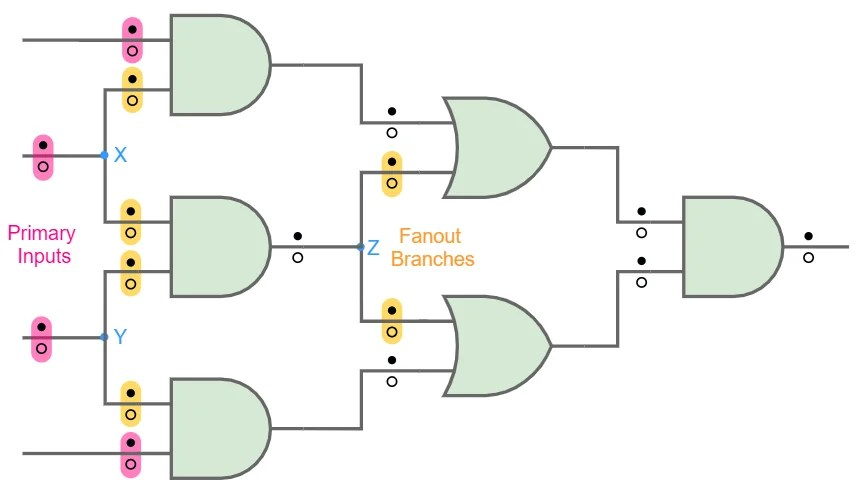## Conclusion

Considering single stuck-at faults we need not test each fault in every wire in the circuit. Most of these faults can be collapsed using equivalence and dominance relations. We necessarily only need to test the checkpoints in the circuit. The checkpoint theorem guarantees 100% fault coverage, but only for combinational circuits.

In the next article, we will consider few structured DFT techniques which will facilitate the testing process for sequential circuits.

Related courses for this will be up soon!

This site uses Akismet to reduce spam. Learn how your comment data is processed.

Top